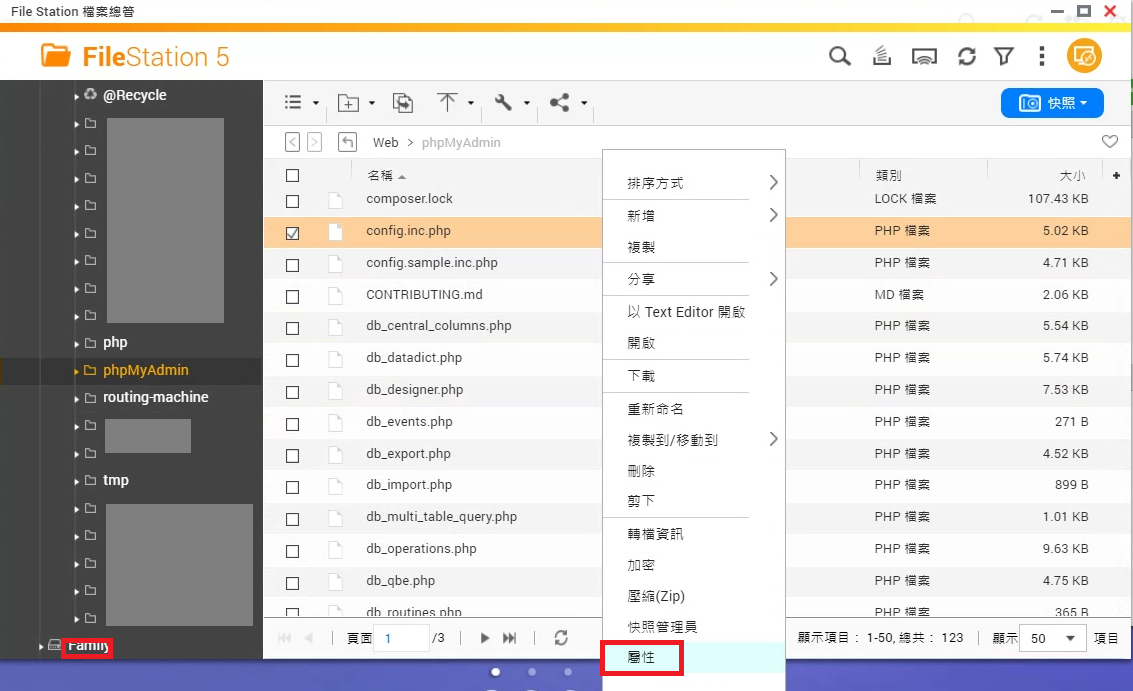## Tuesday, September 13, 2022

### Swift call C++ code and class

[Reference]

https://stackoverflow.com/questions/35229149/interacting-with-c-classes-from-swift

https://stackoverflow.com/questions/2045774/developing-c-wrapper-api-for-object-oriented-c-code/2045860

Swift can not call C++ code directly. C++ code must be wrapped by C, Objective-C or Objective-C++. Swift uses the wrapper (wrapped code) to call C++ code. According to the references, an example is shown as the following:

``````#pragma once
class MPoint3
{
public:
double x;
double y;
double z;
};
``````

``````#ifndef MPoint3C_h
#define MPoint3C_h

#ifdef __cplusplus
extern "C" {
#endif

typedef struct
{
double x;
double y;
double z;
} MPoint3C;

MPoint3C* MPoint3_Constructor(void);
void MPoint3_Destructor(MPoint3C*);

#ifdef __cplusplus
}
#endif

#endif
``````

C++ source (MPoint3C.cpp)

``````#include "MPoint3.hpp"
#include "MPoint3C.h"

#ifdef __cplusplus
extern "C" {
#endif

MPoint3C* MPoint3_Constructor(void)
{
return (MPoint3C*) new MPoint3;
}

void MPoint3_Destructor(MPoint3C* ppt)
{
delete (MPoint3*)ppt;
}

#ifdef __cplusplus
}
#endif
``````

Use in Swift (swift.swift)

``````let pPt = MPoint3_Constructor()   // The type of pPt is UnsafeMutablePointer<MPoint3C>

pPt.pointee.x = 10
pPt.pointee.y = 11
pPt.pointee.z = 12

MPoint3_Destructor(pPt)
``````